# HSSlive: Plus One & Plus Two Notes & Solutions for Kerala State Board

## AP Board Class 8 Maths Chapter 7 Frequency Distribution Tables and Graphs InText Questions Textbook Solutions PDF: Download Andhra Pradesh Board STD 8th Maths Chapter 7 Frequency Distribution Tables and Graphs InText Questions Book AnswersAP Board Class 8 Maths Chapter 7 Frequency Distribution Tables and Graphs InText Questions Textbook Solutions PDF: Download Andhra Pradesh Board STD 8th Maths Chapter 7 Frequency Distribution Tables and Graphs InText Questions Book Answers

## Andhra Pradesh State Board Class 8th Maths Chapter 7 Frequency Distribution Tables and Graphs InText Questions Books Solutions

 Board AP Board Materials Textbook Solutions/Guide Format DOC/PDF Class 8th Subject Maths Chapters Maths Chapter 7 Frequency Distribution Tables and Graphs InText Questions Provider Hsslive

## How to download Andhra Pradesh Board Class 8th Maths Chapter 7 Frequency Distribution Tables and Graphs InText Questions Textbook Solutions Answers PDF Online?

2. Click on the Andhra Pradesh Board Class 8th Maths Chapter 7 Frequency Distribution Tables and Graphs InText Questions Answers.
3. Look for your Andhra Pradesh Board STD 8th Maths Chapter 7 Frequency Distribution Tables and Graphs InText Questions Textbooks PDF.
4. Now download or read the Andhra Pradesh Board Class 8th Maths Chapter 7 Frequency Distribution Tables and Graphs InText Questions Textbook Solutions for PDF Free.

## AP Board Class 8th Maths Chapter 7 Frequency Distribution Tables and Graphs InText Questions Textbooks Solutions with Answer PDF Download

Find below the list of all AP Board Class 8th Maths Chapter 7 Frequency Distribution Tables and Graphs InText Questions Textbook Solutions for PDF’s for you to download and prepare for the upcoming exams:

### 8th Class Maths 7th Lesson Frequency Distribution Tables and Graphs InText Questions and Answers

Do this

Question 1.
Here are the heights of some of Indian cricketers. Find the median height of the team. [Page No. 154]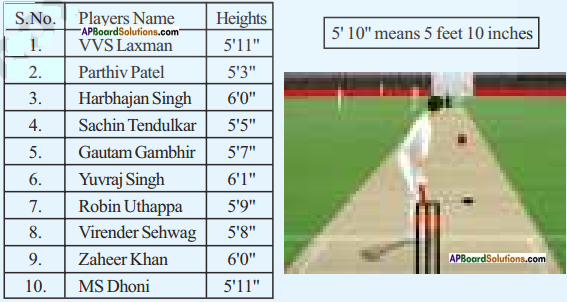The ascending order of heights is 5’3″, 5’5″, 57″, 5’8″, 5’9″, 571″, 571″, 6’0″, 6’0″, 6’7″
Number of players = 10 (is an even)
Median = Mean of (n2) and (𝑛2+1) terms = Mean of (102) and (102+1) terms = Mean of 5, 6 terms =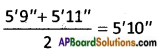Question 2.
Ages of 90 people in an apartment are given in the adjacent grouped frequency distribution.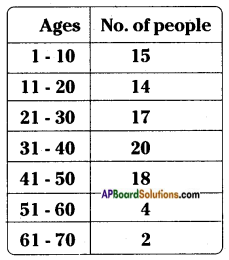i) How many Class Intervals are there in the table?
ii) How many people are there in the Class Interval 21 – 30?
iii) Which age group people are more in that apartment?
iv) Can we say that both people the last age group (61-70) are of 61, 70 or any other age? [Page No. 158]
i) 7 ii) 17 iii) 31 – 40 iv) Yes, they are 62, 63, ……, 69

Question 3.
Long jump made by 30 students of a class are tabulated as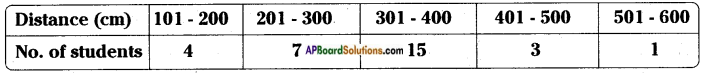I. Are the given class intervals inclusive or exclusive?
II. How many students are in second class interval?
III. How many students jumped a distance of 3.01 m or more?
IV. To which class interval does the student who jumped a distance of 4.005 m belongs? [Page No. 160]
I. Inclusive
II. 7
III. 15 + 3 + 1 = 19
IV. 401 – 500

Question 4.
Calculate the boundaries of the class intervals in the above table. [Page No. 160]
Boundaries:
100.5 – 200.5
2005 – 300.5
300.5 – 400.5
400.5 – 500.5
500.5 – 600.5

Question 5.
What is the length of each class interval in the above table? [Page No. 160]
100

Question 6.
Construct the frequency polygons of the following frequency distributions. [Page No. 174]
i) Runs scored by students of a class in a cricket friendly match.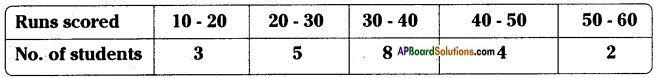ii) Sale of tickets for drama in an auditorium.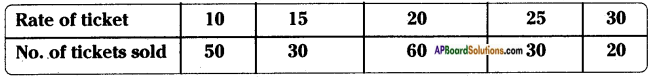i)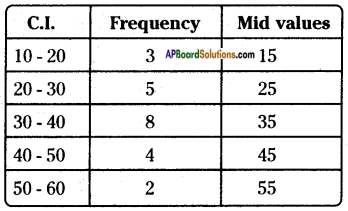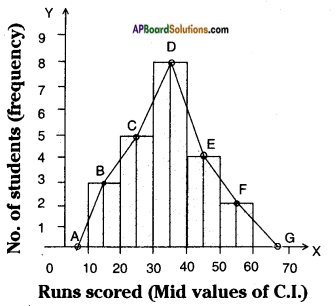Steps of construction: Runs scored (Mid values of C.I.)
Step – 1: Calculate the mid points of every class interval given in the data.
Step – 2: Draw a histogram for this data and mark the mid points of the tops of the rectangles, (like B, C, D, E, F respectively).
Step – 3: Join the mid points successively.
Step – 4: Assume a class interval before the first class and another after the last class. Also calculate their mid values (A and G) and mark on the axis. (Here, the first class is 10 – 20. So, to find the class preceding 10 – 20, we extend the horizontal axis in the negative direction and find the mid point of the imaginary class interval 60 – 70.
Step – 5: Join the first end point B to A and last end point F to G which completes the frequency polygon.
Frequency polygon can also be drawn independently without drawing histogram. For this, we require the midpoints of the class interval of the data.

ii)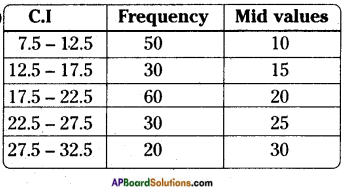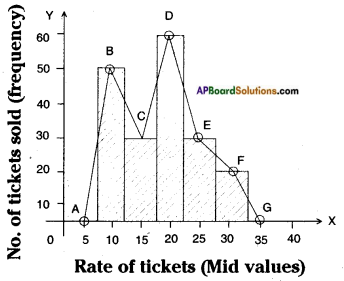Steps of construction:
Step -1: Calculate the mid points of every class interval given in the data.
Step – 2: Draw a histogram for this data and mark the mid points of the tops of the rectangles (like B, C, D, E, F respectively).
Step – 3: Join the mid points successively.
Step – 4: Assume a class interval before the first class and another after the last class.
Step – 5: To find the class preceding 2.5 – 7.5, we extend the horizontal axis in the negative direction and find the mid point of the imaginary class interval 32.5 – 37.5 like A, G.
Step – 6: Join A to B and G to F.
∴ The required ABCDEFG polygon is formed.

Try these

Question 1.
Give any three examples of data which are in situations or in numbers. [Page No. 148]
1) The data of 35 students who like different games: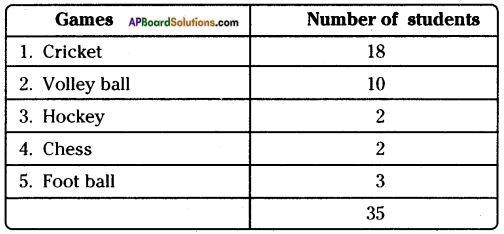2) The data of 35 students who like different colours: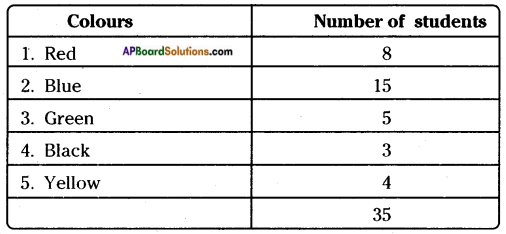3) The data of 35 students who like different fruits: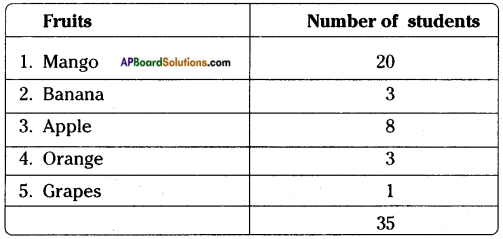Question 2.
Prepare a table of estimated mean, deviations of the above cases. Observe the average of deviations with the difference of estimated mean and actual mean. What do you infer?
[Hint: Compare with average deviations] [Page No. 151]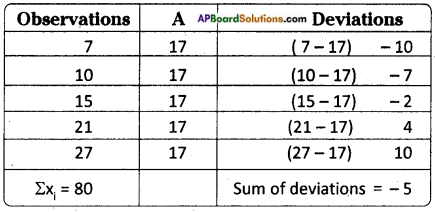Mean = Σ𝑥𝑖𝑁 = 805 = 16
Mean of the deviations = −55 = -1
Mean = Assumed mean + Mean of deviations = 17 + (-1) = 16
∴ Assumed mean, original arithmetic mean are equal.

Question 3.
Estimate the arithmetic mean of the following data. [Page No. 153]
i) 17, 25, 28, 35, 40
ii) 5, 6, 7, 8, 8, 10 10, 10, 12, 12, 13, 19, 19, 19, 20
i) 17, 25, 28, 35, 40
Assumed Mean = 35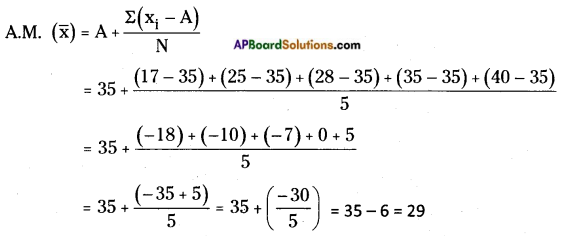A.M in general method =  Sum of the observations  No. of the observations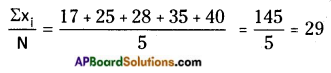ii) 5, 6, 7, 8, 8, 10, 10, 10, 12, 12, 13, 19, 19, 19, 20
Assumed Mean = 10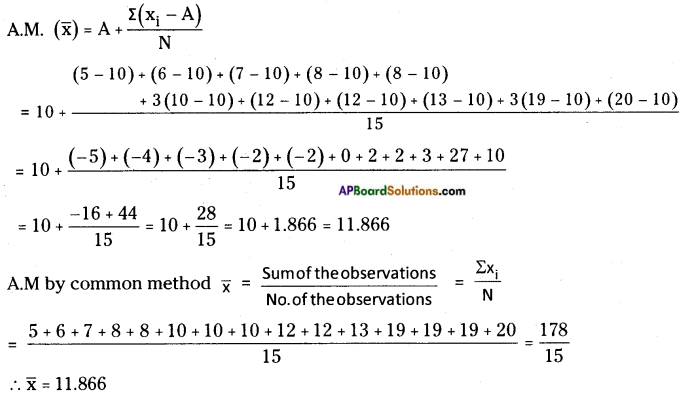Question 4.
Find the median of the data 24, 65, 85, 12, 45, 35, 15. [Page No. 155]
The ascending order of the data is 12, 15, 24, 35, 45, 65, 85
Number of observations (n) = 7 (odd)
∴ Median = 𝑛+12 = 7+12 = 4th term
∴ Median = 35

Question 5.
If flie median of x, 2x, 4x is 12, then find mean of the data. [Page No. 155]
Given observations are x, 2x, 4x
∴ Median = 2x
According to the sum
2x = 12 ⇒ x = 6
2x = 2 × 6 = 12
4x = 4 × 6 = 24
∴ The mean of 6, 12, 24 = 6+12+243 = 423 = 14

Question 6.
If the median of the data 24, 29, 34, 38, x is 29 then the value of ‘x’ is
i) x > 38 ii) x < 29 iii) x lies in between 29 and 34 iv) none [Page No. 155]
Median of 24, 29, 34, 38, x is 29.
n = 5 is an odd.,
∴ Median = 𝑛+12 = 5+12 = 3rd term
If x is less than 29, then only 29 should be a 3rd term.
∴ x < 29

Question 7.
Less than cumulative frequency is related to ……. [Page No. 165]
Upper boundaries

Question 8.
Greater than cumulative frequency is related to …….. [Page No. 165]
Lower boundaries

Question 9.
Write the Less than and Greater than cumulative frequencies for the following data. [Page No. 165]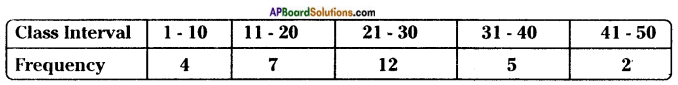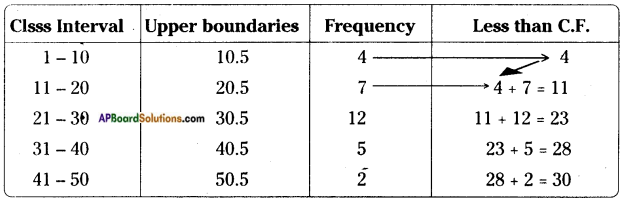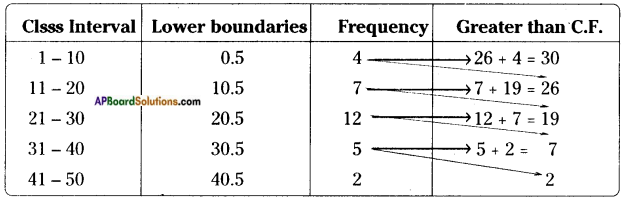Question 10.
What is total frequency and less than cumulative frequency of the last class above problem? What do you infer? [Page No. 165]
The sum of the frequencies in the above distribution table = 30
Less than C.F of the last C.I = 30
∴ Sum of the observations = Less than C.F of last C.I.

Question 11.
Observe the adjacent histogram and answer the following questions. [Page No. 169]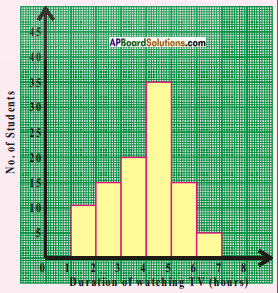i) What information is being represented in the histogram?
ii) Which group contains maximum number of students?
iii) How many students watch TV for 5 hours or more?
iv) How many students are surveyed in total?
i) The histogram represents students who watch the T.V.’s .(Duration of watching T.V).
ii) 4th class interval contains maximum number of students.
iii) 35 + 15 + 5 = 55
iv) Number of students are surveyed = 10 + 15 + 20 + 35 + 15 + 5 = 100

Think, discuss and write

Question 1.
Is there any change in mode, if one or two or more observations, equal to mode are included in the data? [Page No. 155]
If one or two or more observations equal to mode are included there will be no change in the mode.
Ex: The mode of 5, 6, 7, 8, 7, 9 is 7.
If 3, 7’s are added to above observations there will be no change in the mode.

Question 2.
Make a frequency distribution of the following series.
1, 2, 2, 3, 3, 3, 3, 3, 4, 4, 4, 4, 4, 4, 4, 4, 4, 5, 5, 5, 5, 5, 5, 5, 6, 6, 6, 6, 7, 7. [Page No. 161]
The range of the observations = Highest value – Least value
∴ Range = 7 – 1 = 6
If number of classes = 7 then
Class Interval =  Range  No. of classes  = 67 = 0.8 (approx.)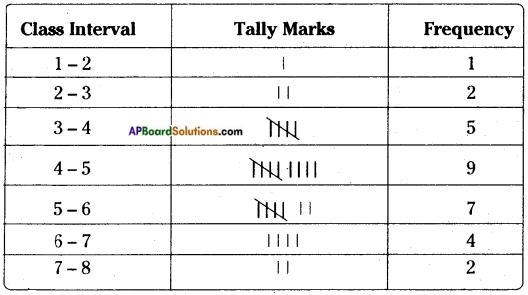Question 3.
Construct a frequency distribution for the following series of numbers.
2, 3, 4, 6, 7, 8, 9, 9, 11, 12, 12, 13, 13, 13, 14, 14, 14, 15, 16, 17, 18, 18, 19, 20, 20, 21, 22, 24, 24, 25. (Hint: Use inclusive classes) [Page No. 161]
Range = Maximum value – Minimum value = 25 – 2 = 23
Class Interval =  Range  No. of classes  = 235 = 4.6 = 5 (approx.) [∵ No. of classes = 5]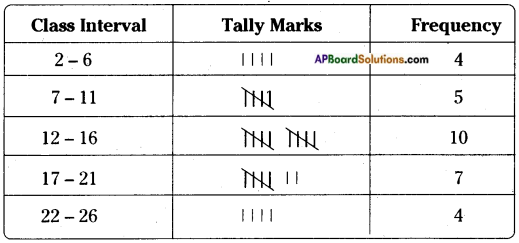Question 4.
What are the differences between the above two frequency distribution tables? [Page No. 161]
The class intervals of first frequency distribution table are exclusive class intervals. The C.I’s of 2nd frequency distribution table are inclusive class intervals.

Question 5.
From which of the frequency distributions we can write the raw data again? [Page No. 161]
Classes

Question 6.
All the bars (or rectangles) in a bar graph have [Page No. 168]
a) same length b) same width c) same area d) equal value
b) same width

Question 7.
Does the length of each bar depend on the lengths of other bars in the graphs? [Page No. 168]
No

Question 8.
Does the variation in the value of a bar affect the values of other bars in the same graph? [Page No. 168]
No

Question 9.
Where do we use vertical bar graphs and horizontal bar graphs? [Page No. 168]
Vertical and horizontal bar graphs are used to present the equal widths corresponding to the given frequencies.

Question 10.
Class boundaries are taken on the X-axis. Why not class limits? [Page No. 172]
The difference between upper and lower boundaries gives the class interval i.e., we take class boundaries on X-axis.

Question 11.
Which value decides the width of each rectangle in the histogram? [Page No. 172]
Class Interval

Question 12.
What does the sum of heights of all rectangles represent? [Page No. 172]
Sum of the frequencies.

Question 13.
How do we complete the polygon when there is no class preceding the first class? [Page No. 173]
The frequency of preceding class should be taken as ‘0’ (zero) then it should be joined.

Question 14.
The area of histogram of a data and its frequency polygon are same. Reason how? [Page No. 173]
Because both the figures are constructed on the basis of mid values of class intervals.

Question 15.
Is it necessary to draw histogram for drawing a frequency polygon? [Page No. 173]
No need.

Question 16.
Shall we draw a frequency polygon for frequency distribution of discrete series? [Page No. 173]
No, we can’t.

Question 17.
Histogram represents frequency over a class interval. Can it represent the frequency at a particular point value? [Page No. 175]
Yes, the histogram represents the frequency at a particular point value. Since the length of a histogram represents the value of its corresponding frequency (length of the frequency).

Question 18.
Can a frequency polygon give an idea of frequency of observations at a particular point? [Page No. 175]
Yes, we can identify the frequency of observation with a frequency polygon at a particular point. Since the height of the polygon is equal to frequency of polygon

## Andhra Pradesh Board Class 8th Maths Chapter 7 Frequency Distribution Tables and Graphs InText Questions Textbooks for Exam Preparations

Andhra Pradesh Board Class 8th Maths Chapter 7 Frequency Distribution Tables and Graphs InText Questions Textbook Solutions can be of great help in your Andhra Pradesh Board Class 8th Maths Chapter 7 Frequency Distribution Tables and Graphs InText Questions exam preparation. The AP Board STD 8th Maths Chapter 7 Frequency Distribution Tables and Graphs InText Questions Textbooks study material, used with the English medium textbooks, can help you complete the entire Class 8th Maths Chapter 7 Frequency Distribution Tables and Graphs InText Questions Books State Board syllabus with maximum efficiency.

## FAQs Regarding Andhra Pradesh Board Class 8th Maths Chapter 7 Frequency Distribution Tables and Graphs InText Questions Textbook Solutions

#### Can we get a Andhra Pradesh State Board Book PDF for all Classes?

Yes you can get Andhra Pradesh Board Text Book PDF for all classes using the links provided in the above article.

## Important Terms

Andhra Pradesh Board Class 8th Maths Chapter 7 Frequency Distribution Tables and Graphs InText Questions, AP Board Class 8th Maths Chapter 7 Frequency Distribution Tables and Graphs InText Questions Textbooks, Andhra Pradesh State Board Class 8th Maths Chapter 7 Frequency Distribution Tables and Graphs InText Questions, Andhra Pradesh State Board Class 8th Maths Chapter 7 Frequency Distribution Tables and Graphs InText Questions Textbook solutions, AP Board Class 8th Maths Chapter 7 Frequency Distribution Tables and Graphs InText Questions Textbooks Solutions, Andhra Pradesh Board STD 8th Maths Chapter 7 Frequency Distribution Tables and Graphs InText Questions, AP Board STD 8th Maths Chapter 7 Frequency Distribution Tables and Graphs InText Questions Textbooks, Andhra Pradesh State Board STD 8th Maths Chapter 7 Frequency Distribution Tables and Graphs InText Questions, Andhra Pradesh State Board STD 8th Maths Chapter 7 Frequency Distribution Tables and Graphs InText Questions Textbook solutions, AP Board STD 8th Maths Chapter 7 Frequency Distribution Tables and Graphs InText Questions Textbooks Solutions,
Share: